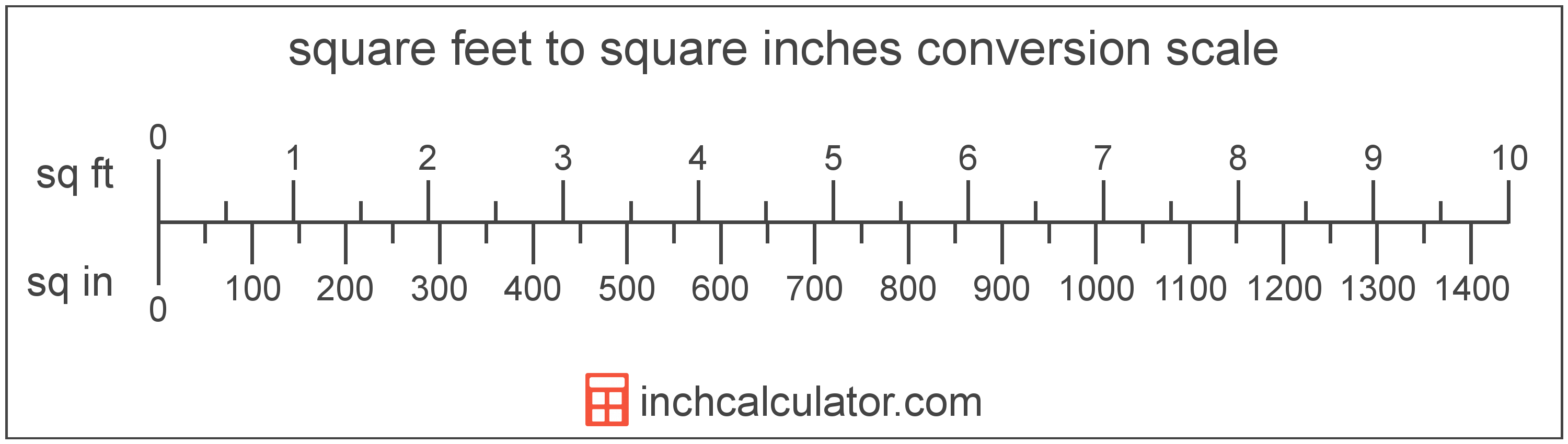# Square Feet to Square Inches Conversion

Enter the area in square feet below to get the value converted to square inches.

(find square feet)
Results in Square Inches:1 sq ft = 144 sq in

## How to Convert Square Feet to Square InchesTo convert a square foot measurement to a square inch measurement, multiply the area by the conversion ratio.

Since one square foot is equal to 144 square inches, you can use this simple formula to convert:

square inches = square feet × 144

The area in square inches is equal to the square feet multiplied by 144.

For example, here's how to convert 5 square feet to square inches using the formula above.
5 sq ft = (5 × 144) = 720 sq in

### How Many Square Inches are in a Square Foot?

There are 144 square inches in a square foot, which is why we use this value in the formula above.

1 sq ft = 144 sq in

## Square Feet

One square foot is equal to the area of a square with sides that are 1 foot in length. One square foot is roughly equivalent to 144 square inches.

The square foot is a US customary and imperial unit of area. A square foot is sometimes also referred to as a square ft. Square feet can be abbreviated as sq ft, and are also sometimes abbreviated as ft². For example, 1 square foot can be written as 1 sq ft or 1 ft².

Try our square footage calculator to calculate the area of a space.

## Square Inches

One square inch is equal to the area of a square with sides that are 1 inch in length.

The square inch is a US customary and imperial unit of area. A square inch is sometimes also referred to as a square in. Square inches can be abbreviated as sq in, and are also sometimes abbreviated as in². For example, 1 square inch can be written as 1 sq in or 1 in².

## Square Foot to Square Inch Conversion Table

Square foot measurements converted to square inches
Square Feet Square Inches
1 sq ft 144 sq in
2 sq ft 288 sq in
3 sq ft 432 sq in
4 sq ft 576 sq in
5 sq ft 720 sq in
6 sq ft 864 sq in
7 sq ft 1,008 sq in
8 sq ft 1,152 sq in
9 sq ft 1,296 sq in
10 sq ft 1,440 sq in
11 sq ft 1,584 sq in
12 sq ft 1,728 sq in
13 sq ft 1,872 sq in
14 sq ft 2,016 sq in
15 sq ft 2,160 sq in
16 sq ft 2,304 sq in
17 sq ft 2,448 sq in
18 sq ft 2,592 sq in
19 sq ft 2,736 sq in
20 sq ft 2,880 sq in
21 sq ft 3,024 sq in
22 sq ft 3,168 sq in
23 sq ft 3,312 sq in
24 sq ft 3,456 sq in
25 sq ft 3,600 sq in
26 sq ft 3,744 sq in
27 sq ft 3,888 sq in
28 sq ft 4,032 sq in
29 sq ft 4,176 sq in
30 sq ft 4,320 sq in
31 sq ft 4,464 sq in
32 sq ft 4,608 sq in
33 sq ft 4,752 sq in
34 sq ft 4,896 sq in
35 sq ft 5,040 sq in
36 sq ft 5,184 sq in
37 sq ft 5,328 sq in
38 sq ft 5,472 sq in
39 sq ft 5,616 sq in
40 sq ft 5,760 sq in

## References

1. Merriam-Webster, square foot, https://www.merriam-webster.com/dictionary/square%20foot